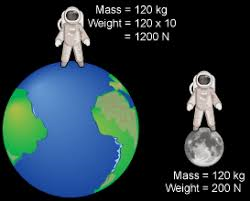## 7th GRADE SCIENCE : Article 19 : Weight and gravity. NEWTON.* Activity 1.29   :   Reviewing balanced forces and Newtons laws.          ( 10/16/17   Monday )

1.29.a    Balanced force wordsearch.     ( 5 points ).

1.29.b    Newtons laws review.     ( 5 points ).

1.29.c    Tug of war balanced force.    ( 5 points ).

* Activity 1.30   :   Halloween writing.                                                   ( 10/17/17   Tuesday )

1.30.a   Halloween writing to spanish students (intercultural Exchange).   ( 10  points ).

* Activity 1.31   :   Working out forces.                                               ( 10/18/17   Wednesday )

1.31.a   Look the following web up :    Work out the following forces.       ( 5 points ).
( Check the example done ).

1.31.b   Now work out the forces at the following data table.        ( 10  points ).

Use your chromebook to creat a table in which you work out
the value of the forces, acceleratios or masses asked.

1.31.c   A truck has a mass of 15,000 kg. The driving force created  by the engine
is 21,000 N. Calculate the acceleration caused by this force.   ( 5 points ).

( You have the solutions there to check).

* Activity 1.32   :   Gravity and weight.                                                    ( 10/19/17   Thursday )

*   These are the questions to answer  looking up the following links :

1.32.a   GRAVITY, MASS AND WEIGHT.

1.32.b   MASS AND WEIGHT.

1.32.c   GRAVITY.

Check your weight on other planets.

Here you can work out mass, gravity or weight.

When you finish answering all the questions.           ( 20 points ).

* Activity 1.33   :   Gravity and weight test.                                                 ( 10/20/17   Friday )

GRAVITY , MASS AND WEIGHT TEST. ( 10  points ).

Newtons laws quizzes.

Comentarios  Ir a formulario